# Cell complex

(diff) ← Older revision | Latest revision (diff) | Newer revision → (diff)
A separable spacethat is a union of non-intersecting cells. Here, by a-dimensional cell one means a topological space that is homeomorphic to the interior of the unit cube of dimension. If for each-dimensional cell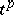ofone is given a continuous mappingfrom the-dimensional cubeintosuch that: 1) the restriction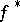ofto the interiorof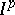is one-to-one and the imageis the closureinof(hereis a homeomorphism ofonto); and 2) the set, where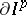is the boundary of, is contained in the union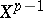of the cells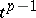of, thenis called a cell complex; the unionis called the skeleton of dimension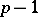of the cell complex. An example of a cell complex is a simplicial polyhedron.
A subset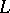of a cell complexis called a subcomplex if it is a union of cells ofcontaining the closures of such cells. Thus, the-dimensional skeletonofis a subcomplex of. Any union and any intersection of subcomplexes ofare subcomplexes of.